Related Articles
Unified Modeling Language (UML) | State Diagrams
• Difficulty Level : Easy
• Last Updated : 07 Nov, 2019

A state diagram is used to represent the condition of the system or part of the system at finite instances of time. It’s a behavioral diagram and it represents the behavior using finite state transitions. State diagrams are also referred to as State machines and State-chart Diagrams. These terms are often used interchangeably. So simply, a state diagram is used to model the dynamic behavior of a class in response to time and changing external stimuli. We can say that each and every class has a state but we don’t model every class using State diagrams. We prefer to model the states with three or more states.

Uses of statechart diagram –

• We use it to state the events responsible for change in state (we do not show what processes cause those events).
• We use it to model the dynamic behavior of the system .
• To understand the reaction of objects/classes to internal or external stimuli.

Firstly let us understand what are Behavior diagrams? There are two types of diagrams in UML :

1. Structure Diagrams – Used to model the static structure of a system, for example- class diagram, package diagram, object diagram, deployment diagram etc.
2. Behavior diagram – Used to model the dynamic change in the system over time. They are used to model and construct the functionality of a system. So, a behavior diagram simply guides us through the functionality of the system using Use case diagrams, Interaction diagrams, Activity diagrams and State diagrams.

Difference between state diagram and flowchart –
The basic purpose of a state diagram is to portray various changes in state of the class and not the processes or commands causing the changes. However, a flowchart on the other hand portrays the processes or commands that on execution change the state of class or an object of the class.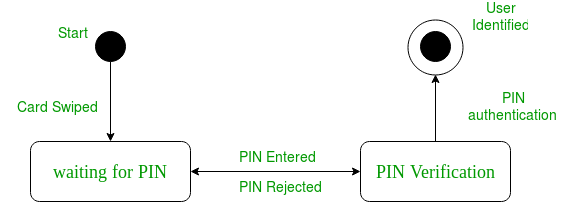Figure – a state diagram for user verification

The state diagram above shows the different states in which the verification sub-system or class exist for a particular system.

### Basic components of a statechart diagram –

1. Initial state – We use a black filled circle represent the initial state of a System or a class.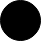Figure – initial state notation
2. Transition – We use a solid arrow to represent the transition or change of control from one state to another. The arrow is labelled with the event which causes the change in state.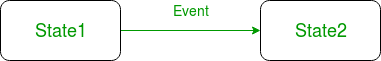Figure – transition
3. State – We use a rounded rectangle to represent a state. A state represents the conditions or circumstances of an object of a class at an instant of time.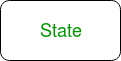Figure – state notation
4. Fork – We use a rounded solid rectangular bar to represent a Fork notation with incoming arrow from the parent state and outgoing arrows towards the newly created states. We use the fork notation to represent a state splitting into two or more concurrent states.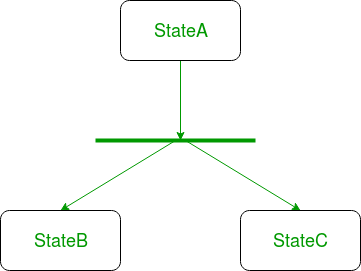Figure – a diagram using the fork notation
5. Join – We use a rounded solid rectangular bar to represent a Join notation with incoming arrows from the joining states and outgoing arrow towards the common goal state. We use the join notation when two or more states concurrently converge into one on the occurrence of an event or events.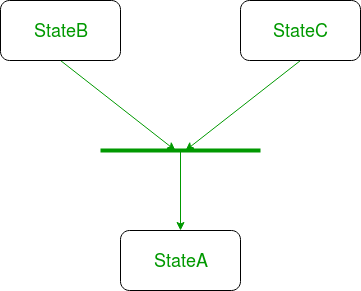Figure – a diagram using join notation
6. Self transition – We use a solid arrow pointing back to the state itself to represent a self transition. There might be scenarios when the state of the object does not change upon the occurrence of an event. We use self transitions to represent such cases.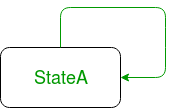Figure – self transition notation
7. Composite state – We use a rounded rectangle to represent a composite state also.We represent a state with internal activities using a composite state.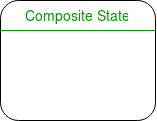Figure – a state with internal activities
8. Final state – We use a filled circle within a circle notation to represent the final state in a state machine diagram.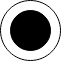Figure – final state notation

### Steps to draw a state diagram –

1. Identify the initial state and the final terminating states.
2. Identify the possible states in which the object can exist (boundary values corresponding to different attributes guide us in identifying different states).
3. Label the events which trigger these transitions.

Example – state diagram for an online order –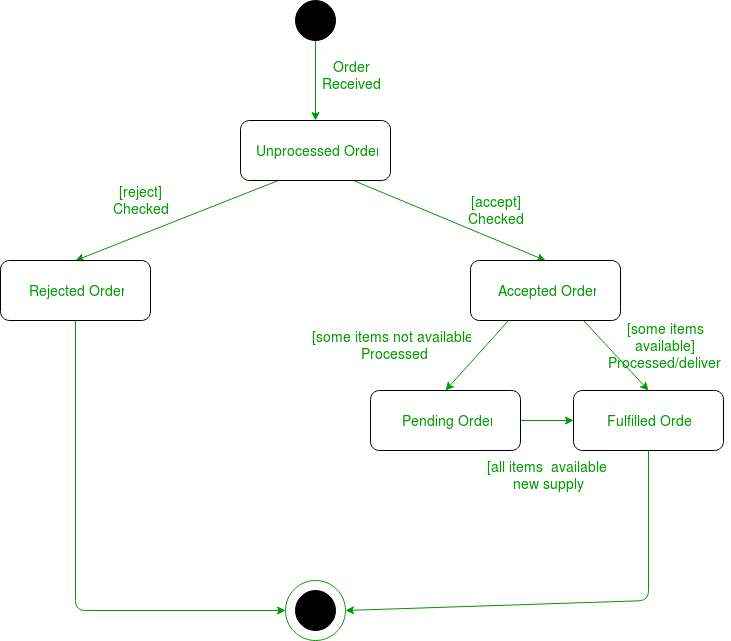Figure – state diagram for an online order

The UMl diagrams we draw depend on the system we aim to represent. Here is just an example of how an online ordering system might look like :

1. On the event of an order being received, we transit from our initial state to Unprocessed order state.
2. The unprocessed order is then checked.
3. If the order is rejected, we transit to the Rejected Order state.
4. If the order is accepted and we have the items available we transit to the fulfilled order state.
5. However if the items are not available we transit to the Pending Order state.
6. After the order is fulfilled, we transit to the final state. In this example, we merge the two states i.e. Fulfilled order and Rejected order into one final state.

Note – Here we could have also treated fulfilled order and rejected order as final states separately.

Reference –
State Diagram – IBM

This article is contributed by Ankit Jain . If you like GeeksforGeeks and would like to contribute, you can also write an article using contribute.geeksforgeeks.org or mail your article to contribute@geeksforgeeks.org. See your article appearing on the GeeksforGeeks main page and help other Geeks.

Please write comments if you find anything incorrect, or you want to share more information about the topic discussed above.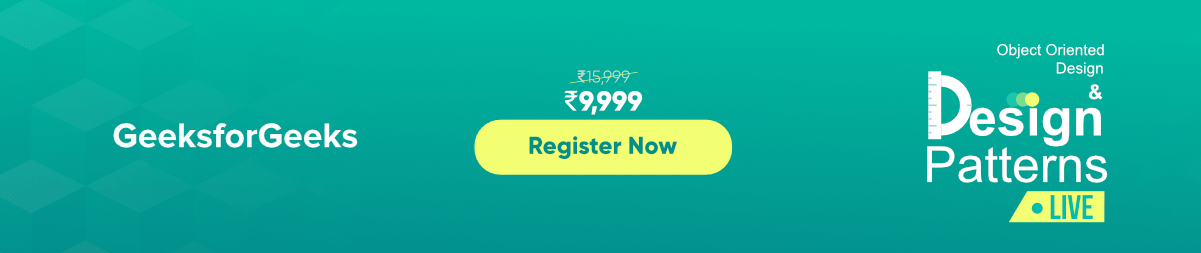My Personal Notes arrow_drop_up